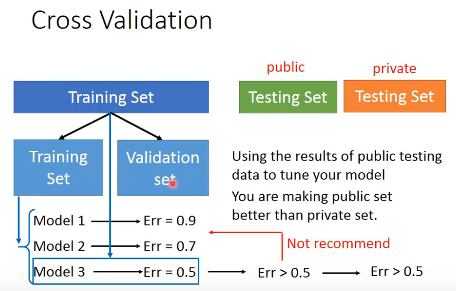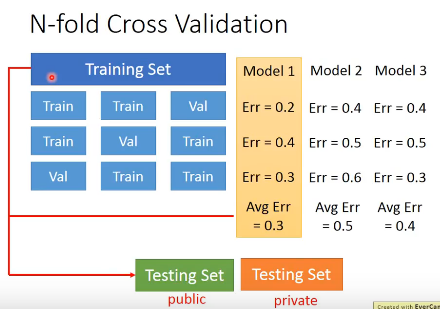2018-05-23 12:01:20 qq_25011449 阅读数 458

# 李宏毅深度学习_Backpropagation

## Background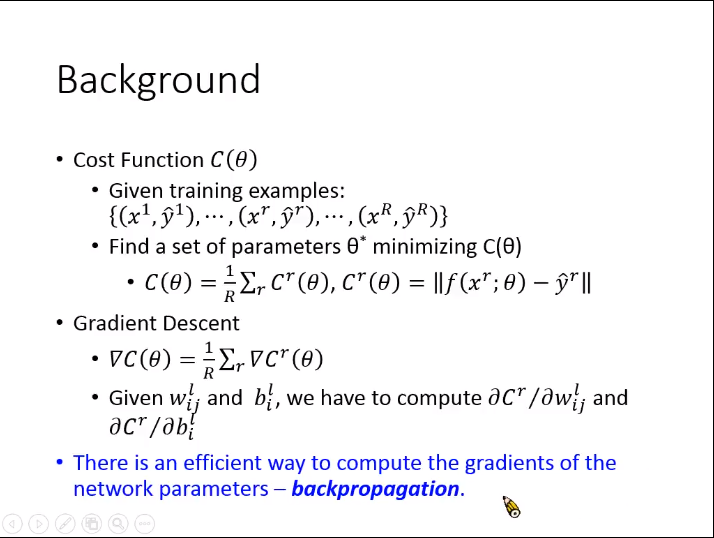## 链式法则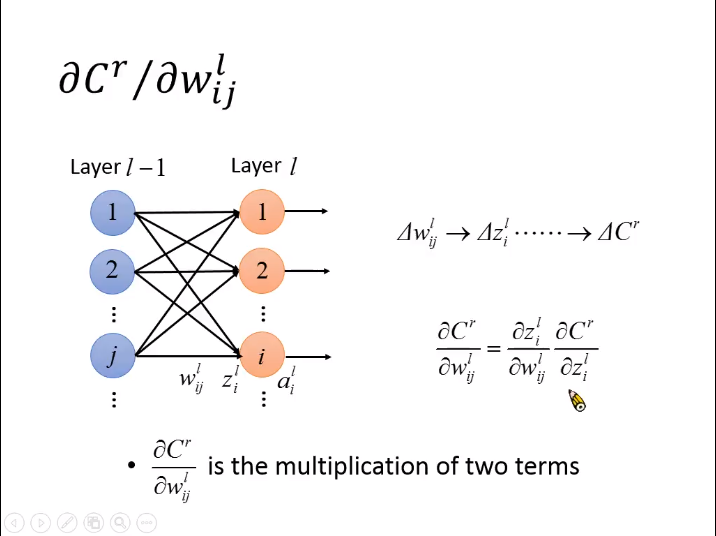## 计算链式法则中拆解的两部分

1. 计算$\mathrm{\partial }{z}_{i}^{l}/\mathrm{\partial }{w}_{ij}^{l}$$\partial z^l_i/ \partial w^l_{ij}$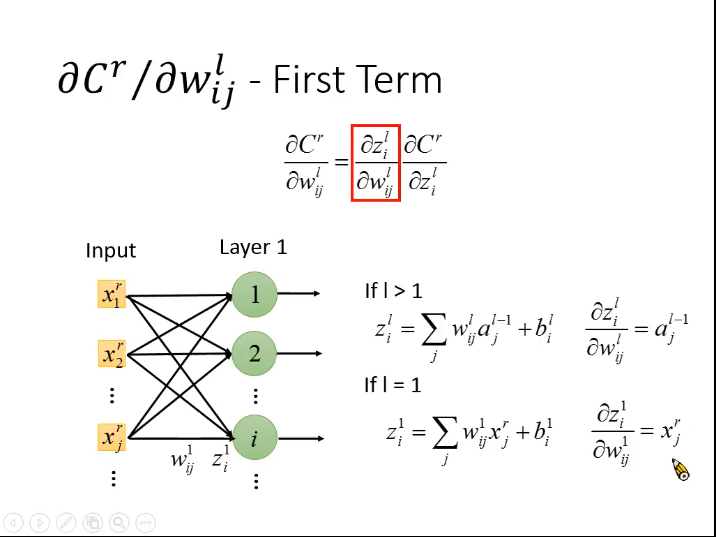• $l>1$$l>1$。即当权值不是第一层时，导数为${a}_{j}^{l-1}$$a^{l-1}_j$
• $l=1$$l=1$。即权值为第一层时，导数为${x}_{j}^{r}$$x^r_j$

2. 计算$\mathrm{\partial }{C}^{r}/\mathrm{\partial }{z}_{i}^{l}$$\partial C^r/ \partial z^l_{i}$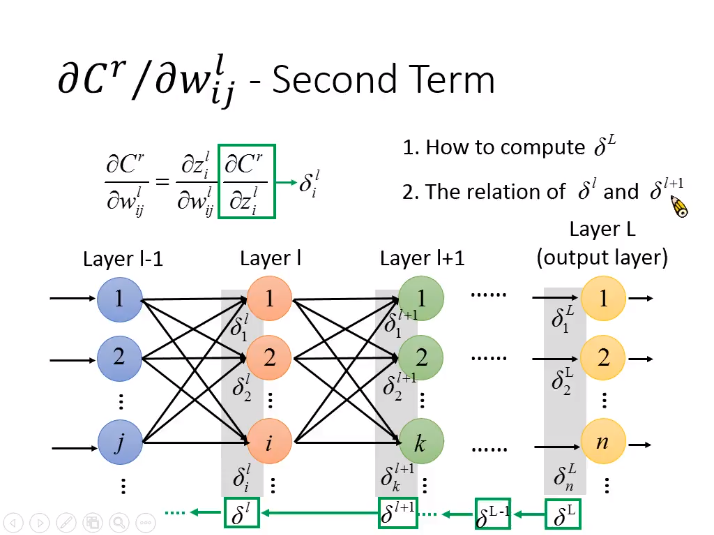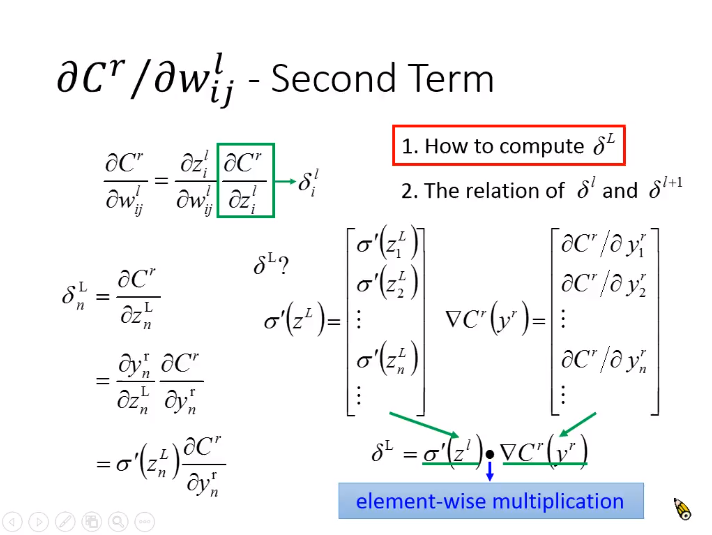${\delta }_{n}^{L}={\sigma }^{\prime }\left({z}_{n}^{L}\right)\frac{\mathrm{\partial }{C}^{r}}{\mathrm{\partial }{y}_{n}^{r}}$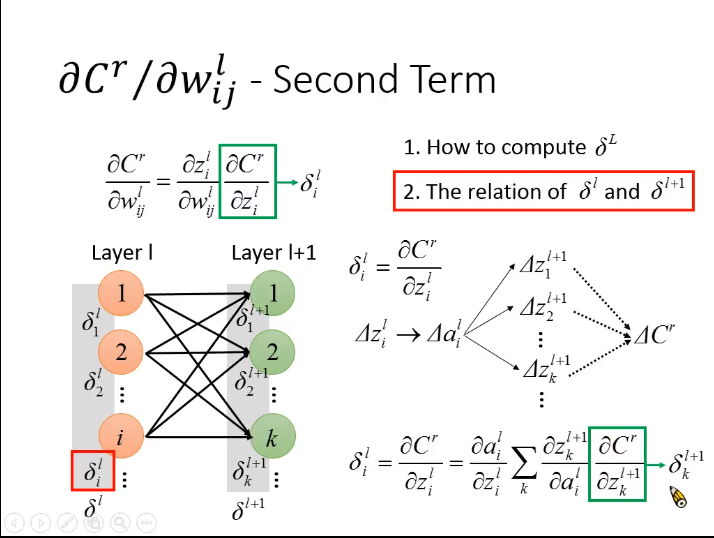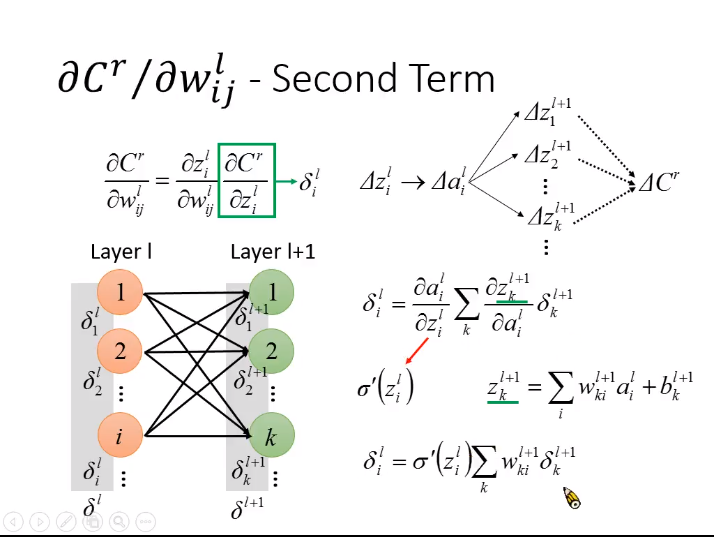${z}_{i}^{l}$$z^l_{i}$的变化$\mathrm{\Delta }{z}_{i}^{l}$$\Delta z^l_{i}$会对${a}_{i}^{l}$$a^l_{i}$造成影响进而影响到下一层的${z}^{l+1}$$z^{l+1}$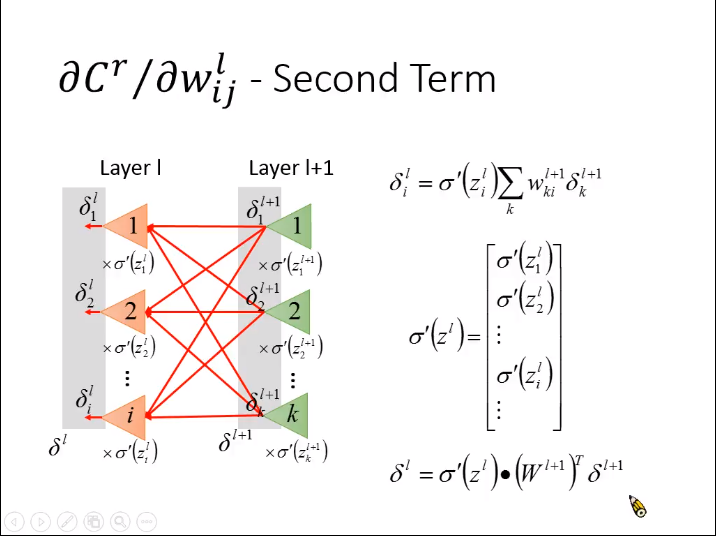${\delta }^{l}={\sigma }^{\prime }\left({z}^{l}\right)\cdot \left({W}^{l+1}{\right)}^{T}{\delta }^{l+1}。$

## 总结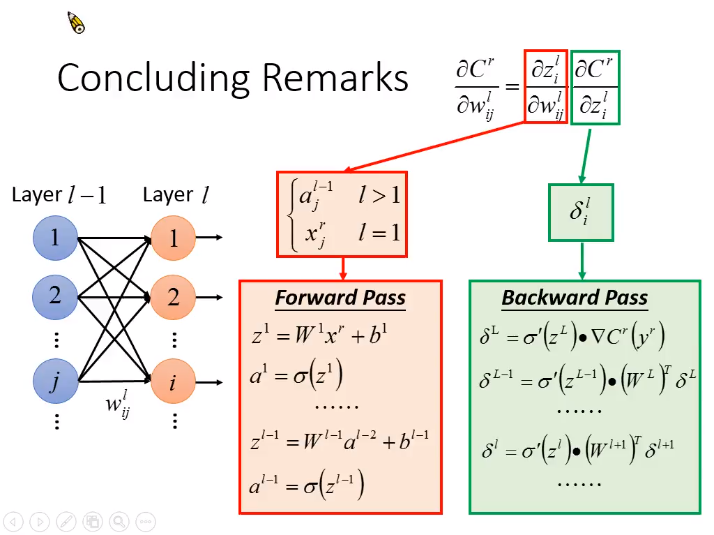2017-11-27 14:42:36 Allenlzcoder 阅读数 2186

# 台大李宏毅2017深度学习国语课程(更新)

本博客转载自：新浪微博爱可可-爱生活
新增SELU、Tuning Hyperparameters、Interesting things about deep learning、Capsule等节，最新#bilibili#完整搬运版：https://www.bilibili.com/video/av9770302/

2019-07-21 15:52:53 weixin_43848117 阅读数 14

# 回归算法的应用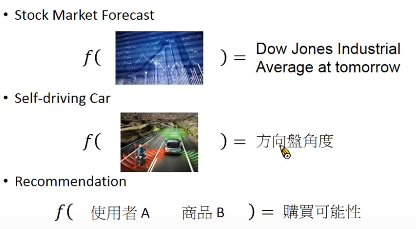## 1.1什么是梯度下降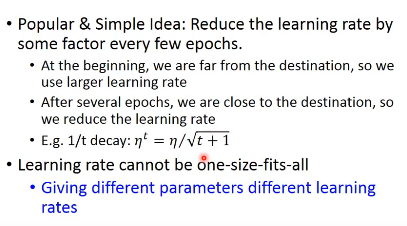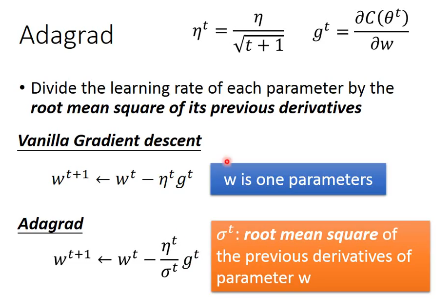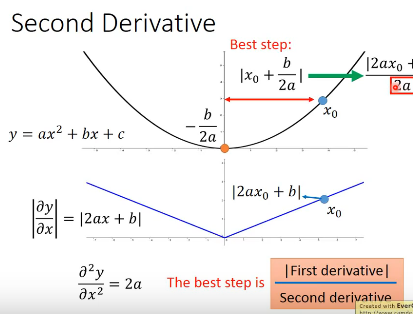## 1.2 随机梯度下降（SGD）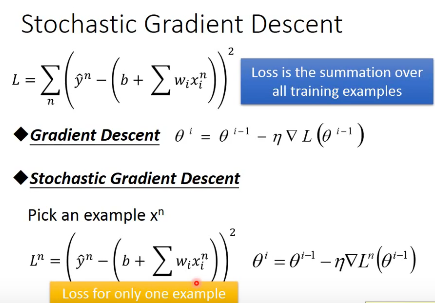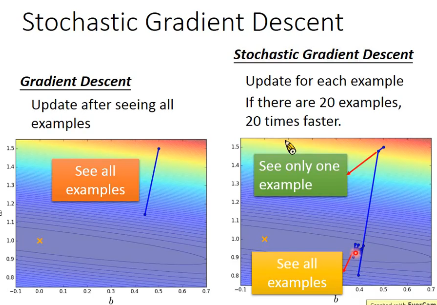## 1.3 特征缩放（feature scaling）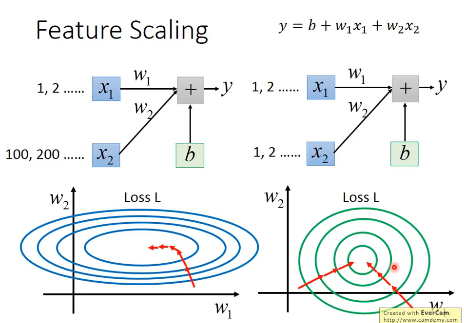### 1.3.1 常用方法—标准化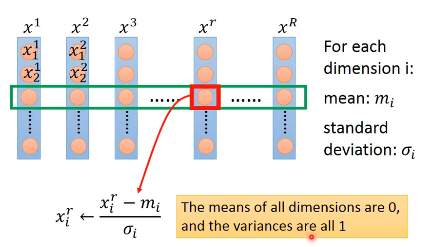## 1.4 梯度下降的限制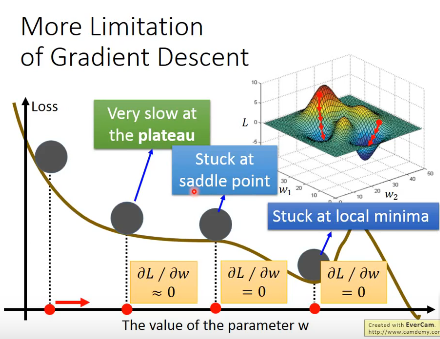2019-08-16 13:56:08 wangwei19871103 阅读数 199

# 深度学习的概况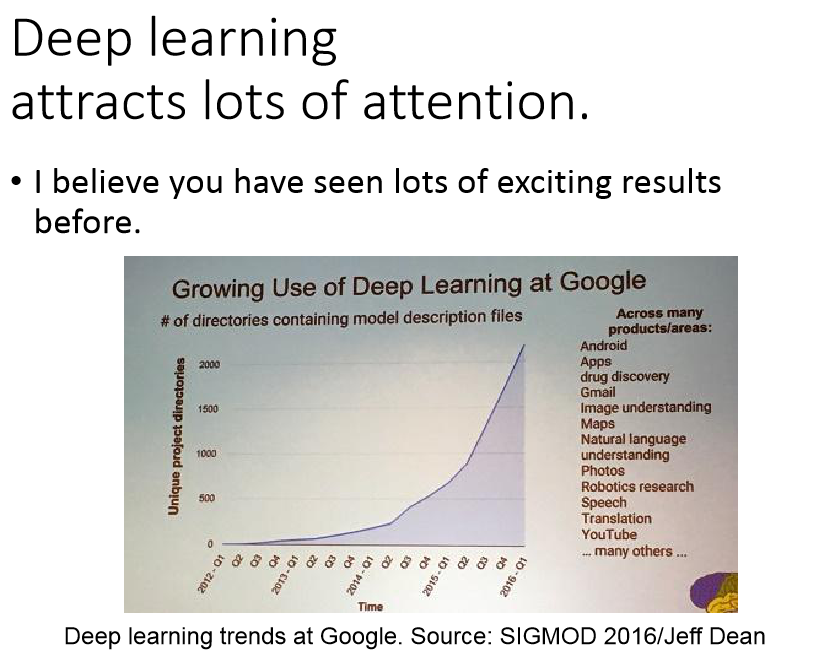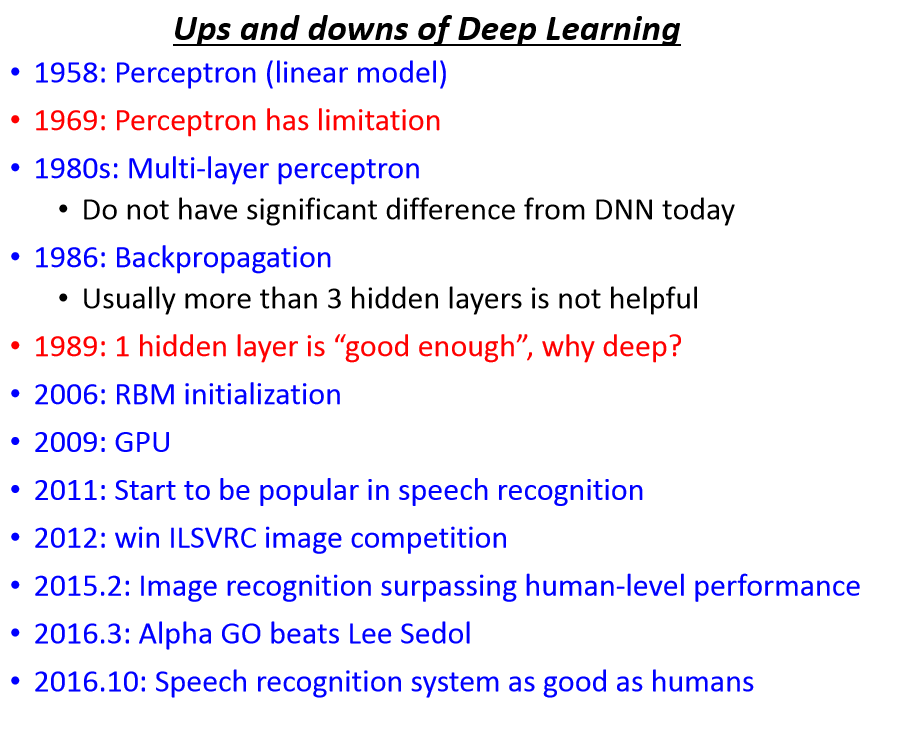# 深度学习的步骤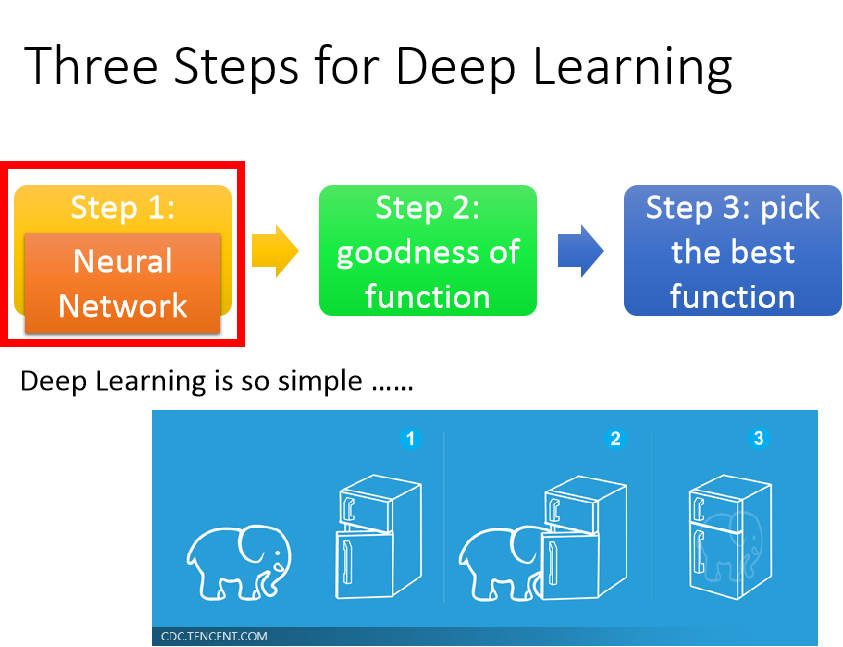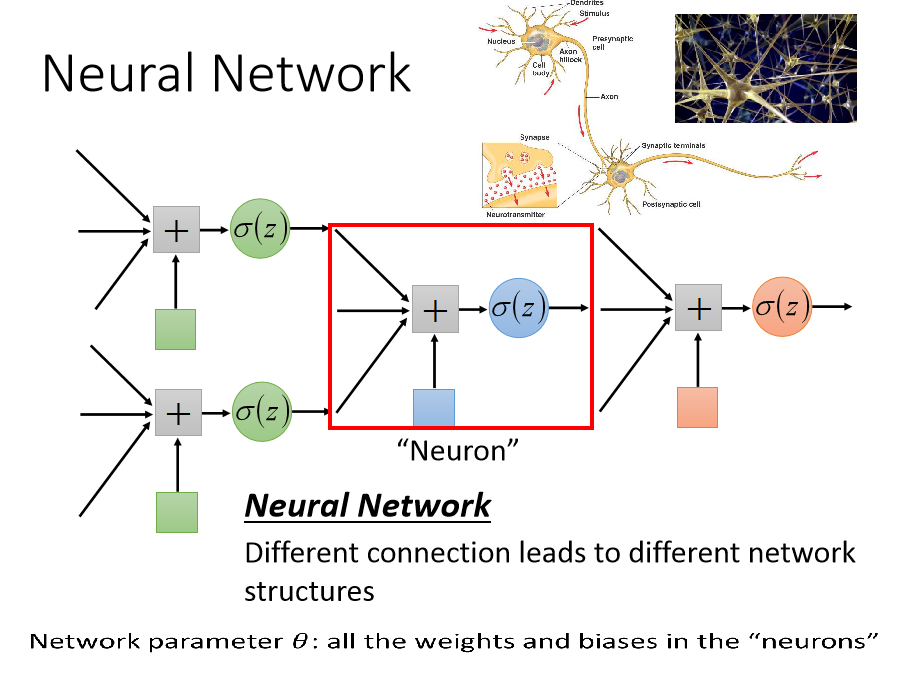# 前馈神经网络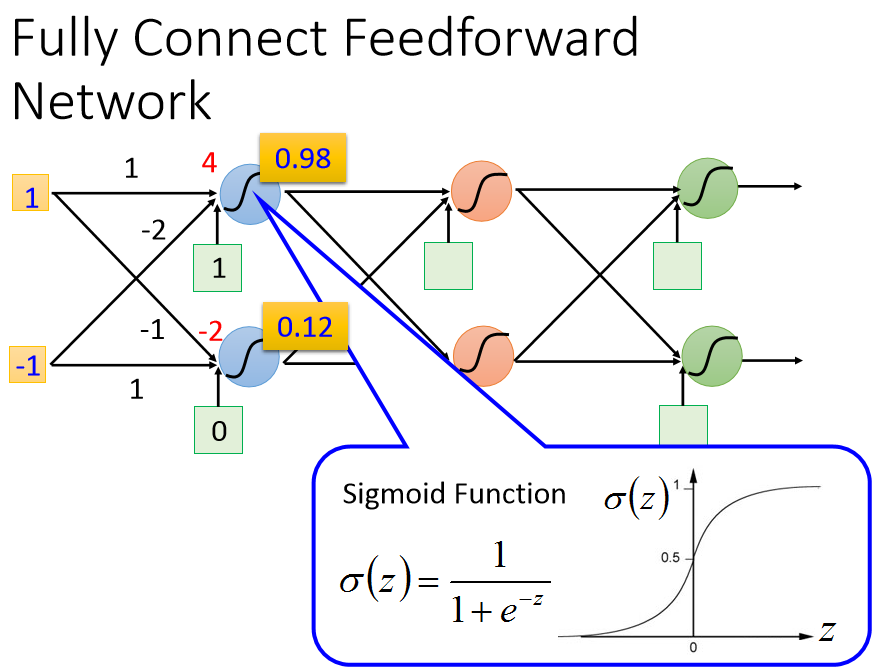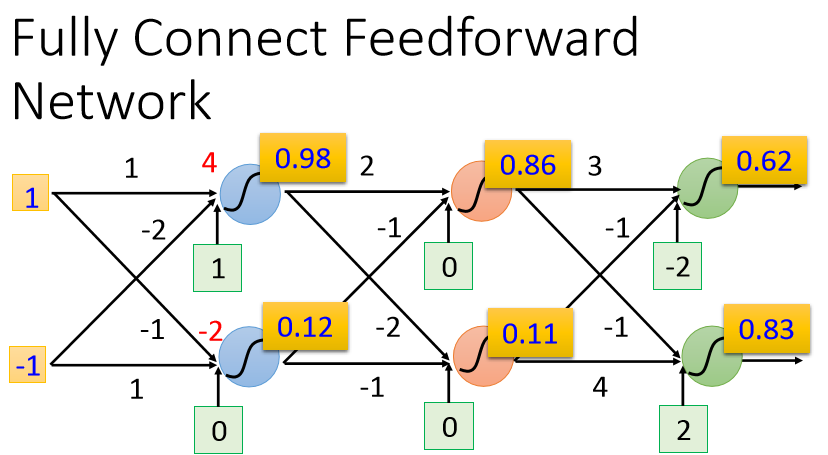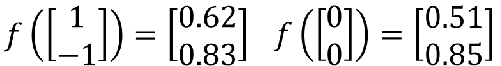# 全连接神经网络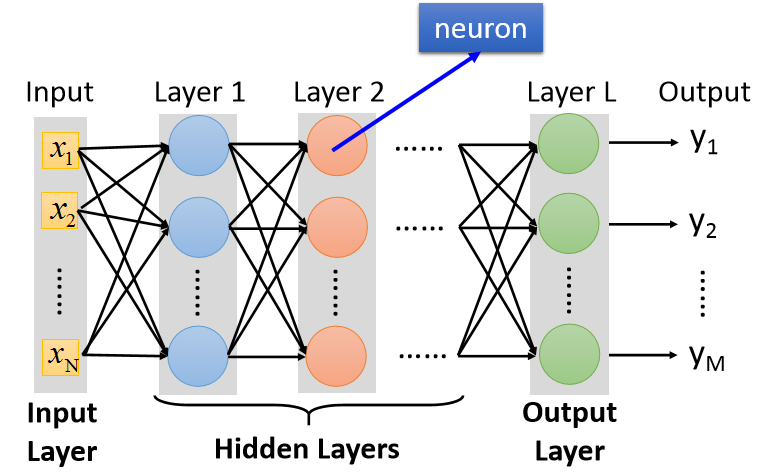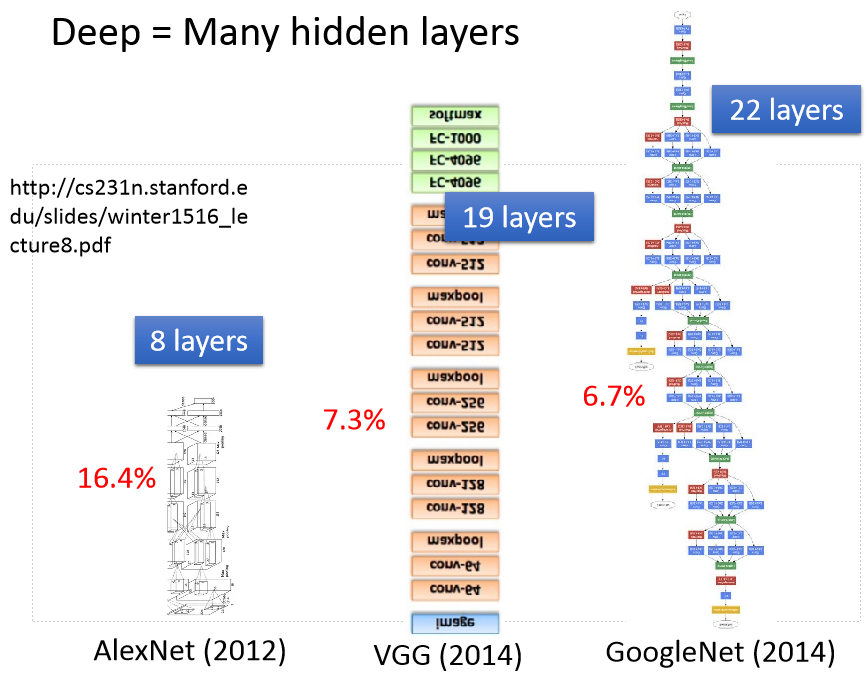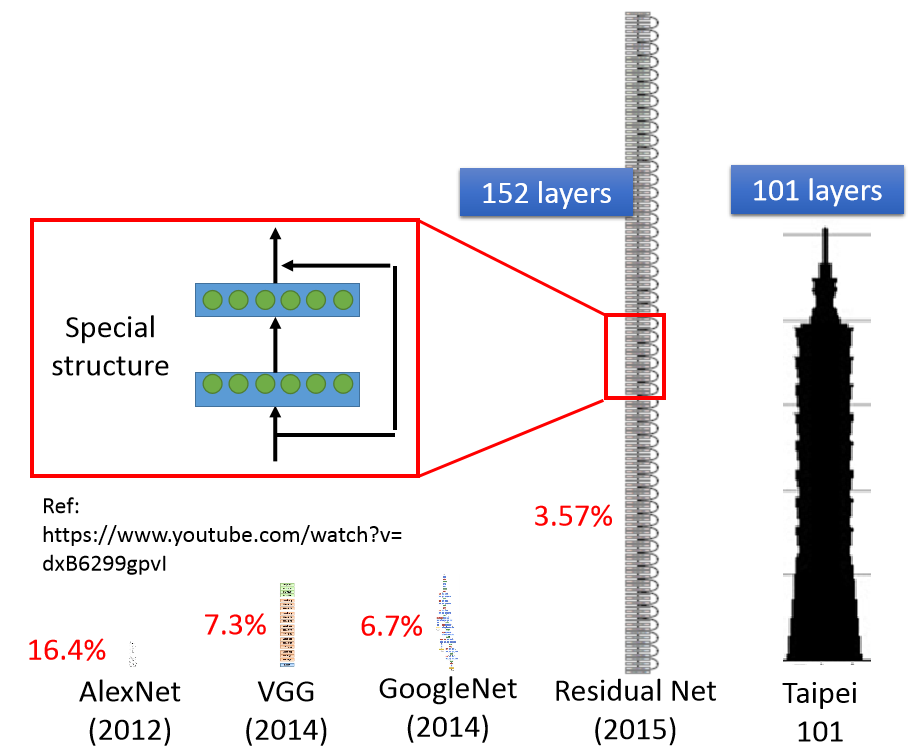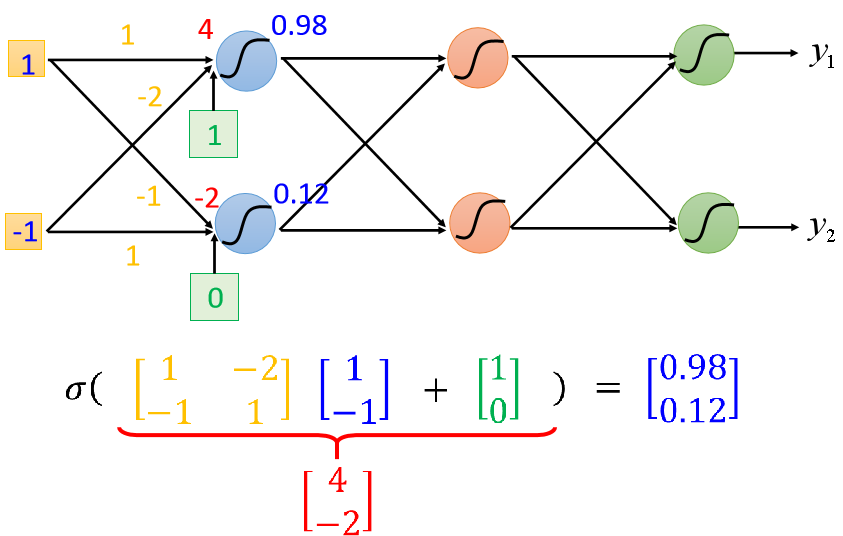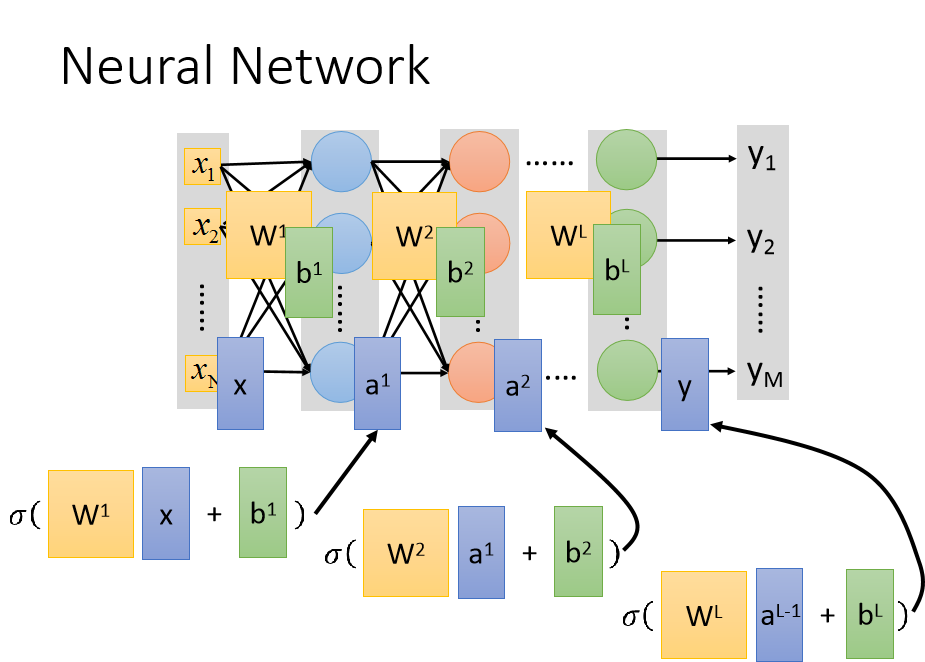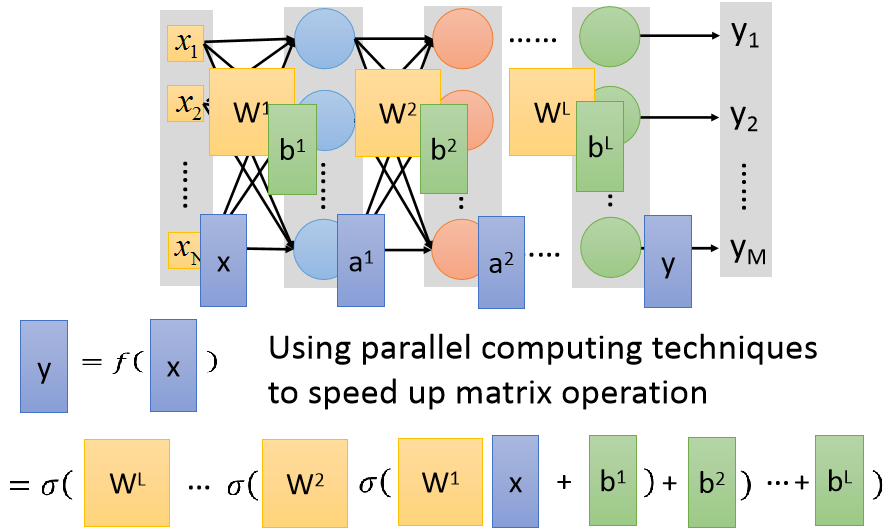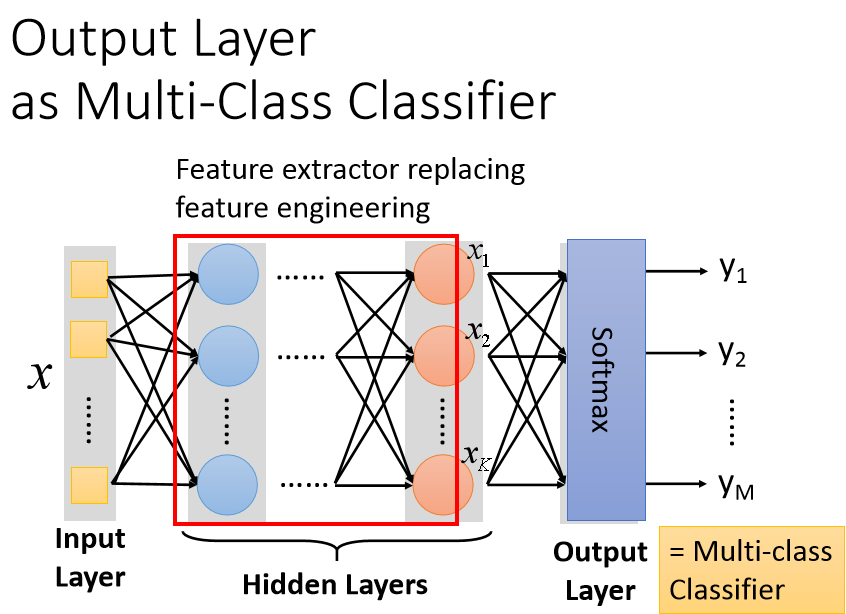# 数字识别分类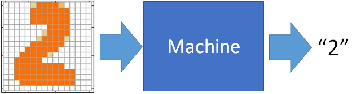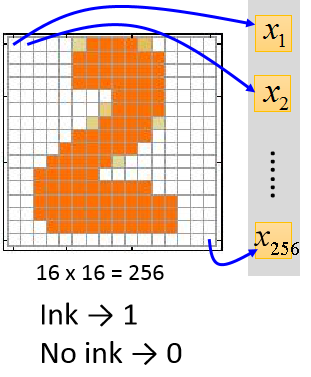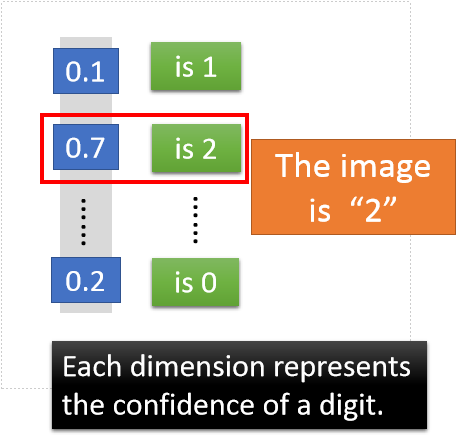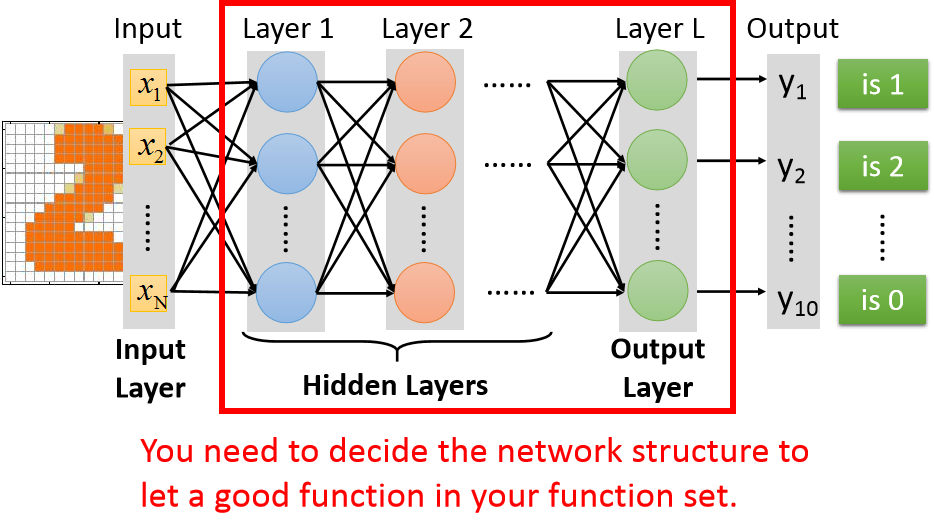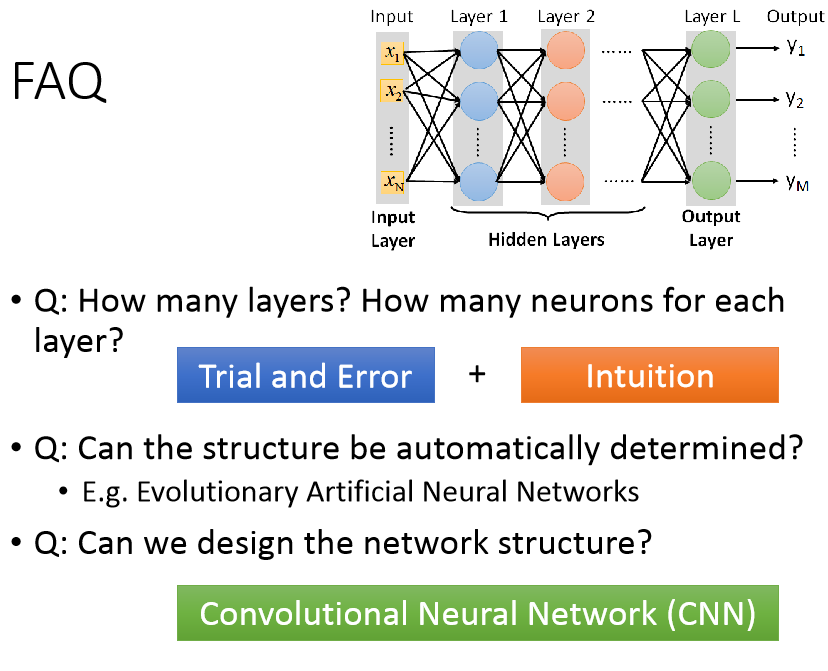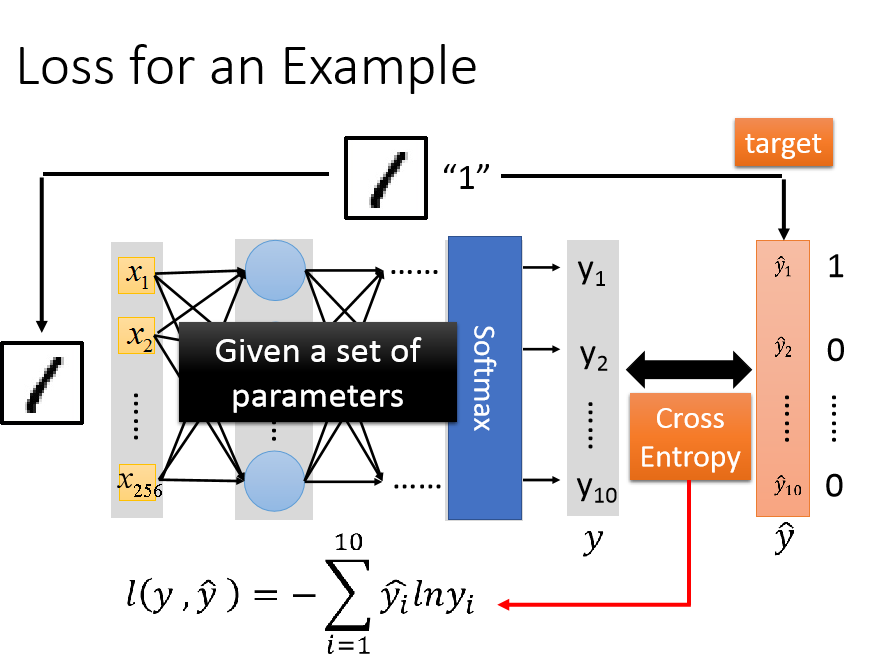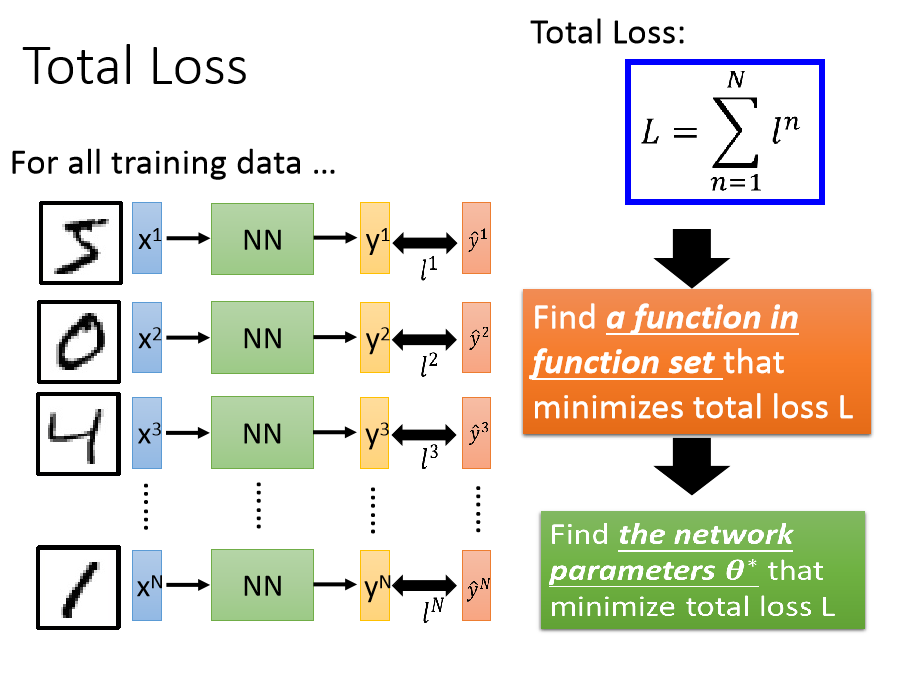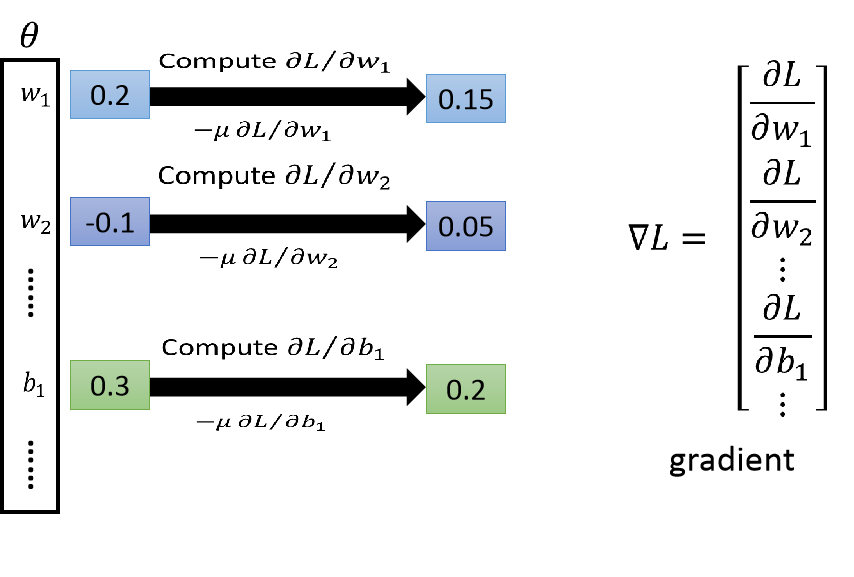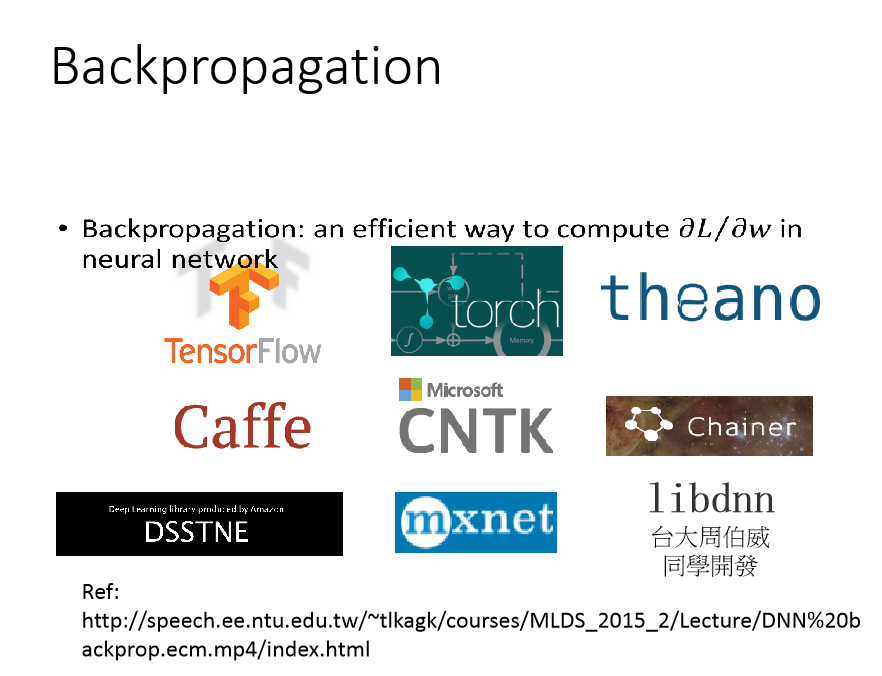# 总结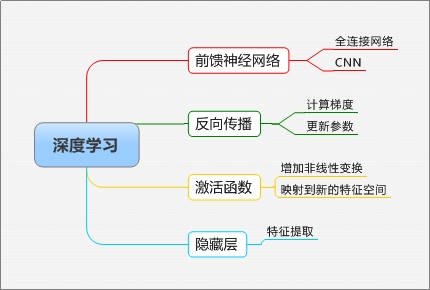2019-07-21 16:46:17 weixin_43848117 阅读数 9

# 1.误差来源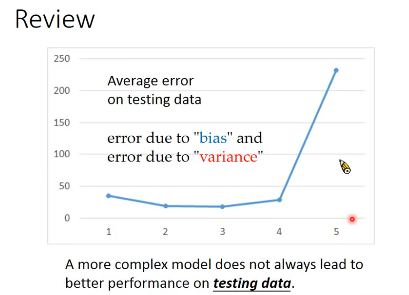## 1.1偏置与方差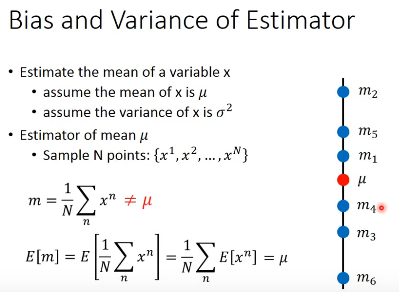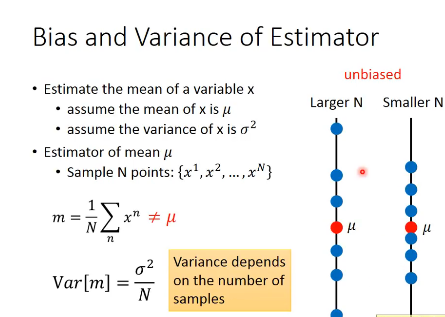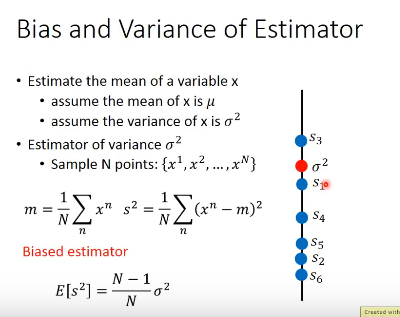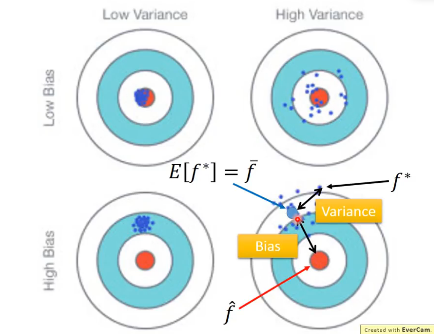## 1.1方差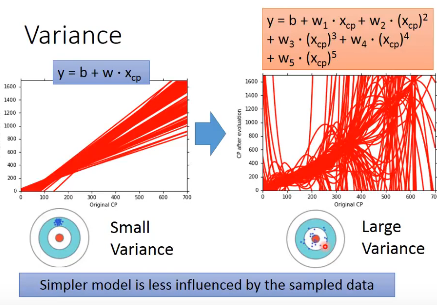## 1.2 偏置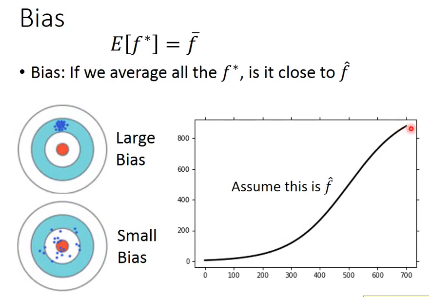## 1.3 模型分析

### 1.3.1模型复杂度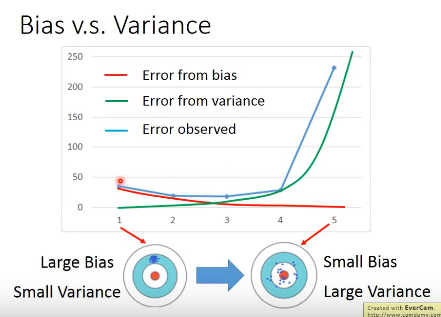### 1.3.2 过拟合、欠拟合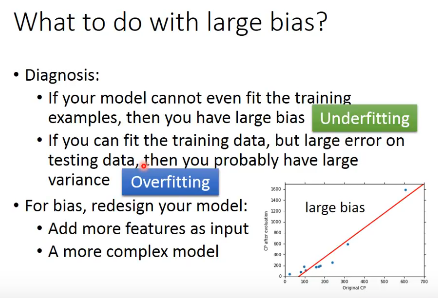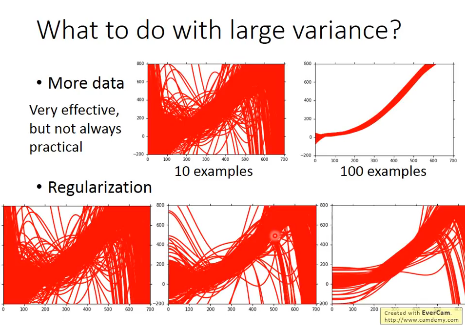# 2.模型选择

## 2.1 模型选择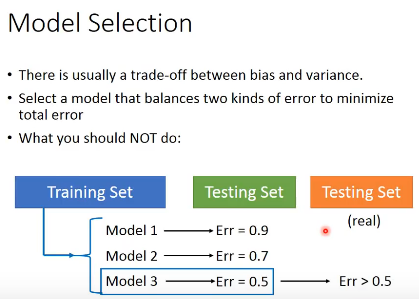## 2.2 模型检测